# What are Silicon Photomultipliers (SiPMs)?

The silicon photomultiplier (SiPM) is a radiation detector with very high sensitivity, extremely low time jitter and high efficiency. It is based on reversed biased p/n diodes and is capable of directly detecting light from near ultra violet to near infrared. SiPMs are used in all those applications where it is necessary for low light/radiation levels to be measured and quantified with high precision.

Light is made up of photons (quantum of light). SiPMs are designed to have high detection efficiency and high gain so that even a single photon impinging on a SiPM pixel can be detected producing an output current pulse with an extremely low time jitter (<100 ps). The structure of a SiPM allows “parallel” photon detection, which means more photons can be detected simultaneously enabling photon counting.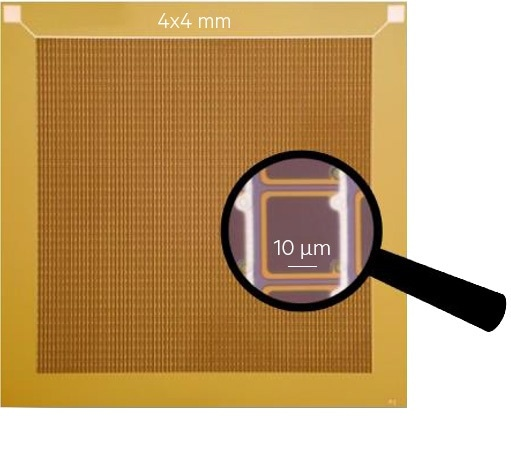Figure 1. Picture of a 4x4 mm2 SiPM die. The SiPM consists of a matrix of micro-cells all connected in parallel. Each micro-cell is a GM-APD and it represents the basic sensitive element of the SiPM. Image Credit: First Sensor

A SiPM comprises of a matrix of small-sized sensitive elements called micro-cells (also known as pixels) all connected in parallel (Figures 1 and 2). Each micro-cell is a Geiger-Mode avalanche photo-diode (GM-APD) functioning beyond the breakdown voltage (Vbd) and it incorporates a resistor for passive quenching.

Figure 3 shows the equivalent circuit of a GM-APD. Cd refers to the diode capacitance in reverse bias, Rs is the silicon substrate series resistance, and Rq is the quenching resistor (Rq >> Rs). There are three central operation modes in a GM-APD: quiescent mode, recovery phase and discharge phase.

In quiescent mode, the diode is reversed biased to Vbias = Vbd +Vov (Vov is the overvoltage,i.e. the excess bias beyond Vbd).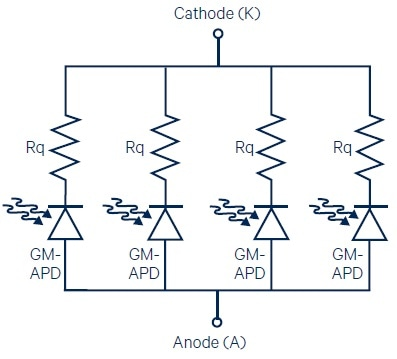Figure 2. The parallel arrangement of GM-APDs with series quenching resistors (Rq) in a SiPM. . Image Credit: First Sensor

The switch in the equivalent circuit of Figure 3 is open and unless a photon is absorbed (or a dark event takes place), the diode continues to be in this condition with no current flowing (neglecting leakage currents). When a photon is absorbed (or a dark event takes place), the equivalent model prescribes that the switch closes and Cd discharges from Vbias to Vbd through Rs: during this phase, avalanche multiplication is ongoing within the GM-APD.

Once triggered, the avalanche process is self-sustaining, which means that without quenching, a stable current flows indefinitely in the device. With Rq, the avalanche process is quenched and the switch in the equivalent circuit of Figure 3 opens again. The GM-APD now enters the recovery phase: Cd recharges back to Vbias through Rq and the GM-APD returns in the quiescent mode, all set for the detection of a new photon.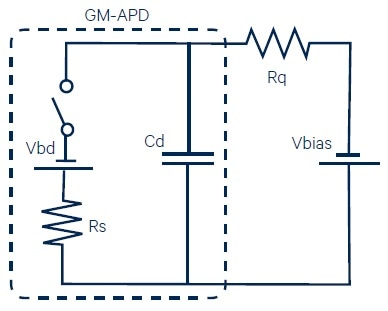Figure 3. The equivalent circuit of a GM-APD with series quenching resistor and external bias. The switch models the turn-on (photon absorption or dark event) and turn-off (quenching) probabilities. . Image Credit: First Sensor

The Cd discharge and recharge occurs at the SiPM terminals as a current pulse (Figure 4). The rising edge matches with the discharge phase (time constant Cd x Rs), whilst the slower trailing edge is the recovery phase with time constant Cd x Rq. To mention that the quenching time is negligible. The amplitude of the SiPM pulse increases with the overvoltage while both the recovery time and the rising time are chiefly determined by Cd, Rq and Rs.

During recovery, the GM-APD will not be able to detect other photons(1). For this reason, it is not possible for a GM-APD to count the number of incoming photons unless the photon rate is lower than the inverse of the recharge time. Thanks to the parallel arrangement of several micro-cells, SiPMs are able to overcome this limitation. When N photons are detected (which means that N photons arrive on N different micro-cells producing N single-cell signals) the SiPM output pulse is N-times greater than the single-cell response. In that, the N independent current pulses simply add up at the SiPM terminals. It should be noted that both the amplitude and the area of each SiPM pulse, which is the total charge delivered by the detector, are proportionate to the number of detected photons (neglecting primary and correlated noise for the time being). If the number of incoming photons is greater than the number of micro-cells in the SiPM, saturation takes place and neither the amplitude nor the area of the output pulse can provide information on the number of incoming photons anymore.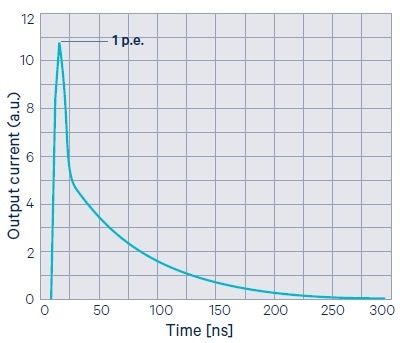Figure 4. Current pulse produced by a micro-cell in response to photon absorption (single-cell signal). The pulse has a very fast rising edge and a slower trailing edge (micro-cell recovery). Its amplitude is defined to be 1 p.e. (photo-electron). Image Credit: First Sensor

In the case of not concurrent cells firing, pile-up of pulses may occur at the SiPM terminals: for example, Figure 6 shows three micro-cells firing in rapid sequence. As before, the area of the SiPM signal is considered to be proportional to the number of the detected photons, but it is less straightforward to recover information from the amplitude of the SiPM signal. The same applies when bunches of simultaneous photons reach the SiPM surface with a specific time distribution: several pulses with varied amplitudes may overlap in time.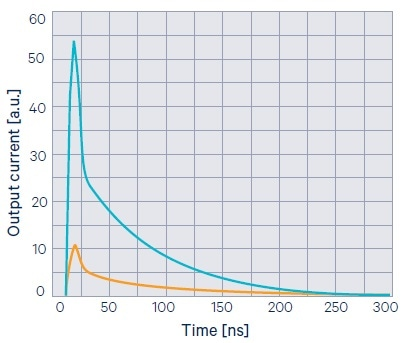Figure 5. Typical SiPM pulse (blue curve) corresponding to simultaneous firing of several cells. The single-cell signal is also reported (orange curve). The SiPM amplitude and pulse area are proportional to the number of detected photons at the same time. . Image Credit: First Sensor

SiPMs can be used for determining the characteristics of the incoming light. For example, when SiPMs are coupled to scintillating crystals, the SiPM output signal mimics, in time, the characteristic light emission profile of the crystal itself (under the notion that the decay time of the crystal is much longer than the recovery time of the SiPM and that the SiPM operates in non-saturation). In an analog way, SiPMs are also used for evaluating the characteristic fluorescence decay time of molecules or substances. By computing the integral of the SiPM signal, the amount of charge produced by the SiPM can be determined, and hence the amount of light emitted by the crystal and eventually the total energy deposited in the crystal, obtaining in this way information on the type (energy) of the radiation being stopped by the crystal (i.e. spectroscopy).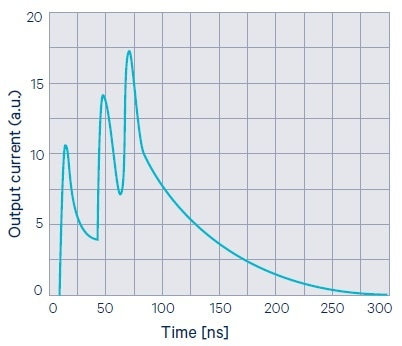Figure 6. Pile-up in a SiPM. Three different micro-cells fire at different times. The SiPM output pulse is the sum of the three single-cell signals. The SiPM signal area (i.e. the total charge delivered by the SiPM) is proportional to the number detected photons.. Image Credit: First Sensor

(1) During recovery the voltage across the GM-APD is increasing from Vbd to (Vbd + Vov), according to the exponential recharge of Cd trough Rq. Consequently, the detection efficiency goes from zero to the value at Vov with the same time constant. The micro-cells are thus not completely blind during recovery; they feature increasing detection efficiency.

## Breakdown Voltage

The breakdown voltage (Vbd) is the minimum (reverse) bias voltage that results in self-sustaining avalanche multiplication in GM-APDs. In other words, it refers to the minimum bias for which output pulses as those shown in Figures 4-6 can be attained. However, for Vbias = Vbd both the detection efficiency and the gain of SiPMs are (by definition) still considered to be null. Only for Vbias > Vbd output current pulses are essentially observed. The excess bias beyond the break-down voltage is known as overvoltage (Vov). By definition:

Vov = Vbias – Vbd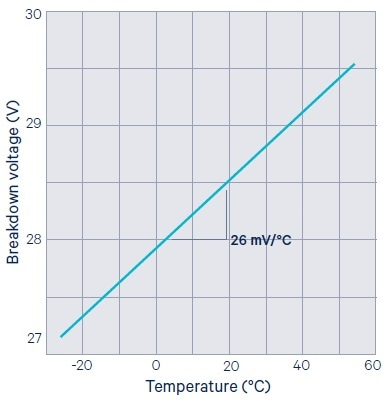Figure 7. Breakdown voltage temperature dependence. The temperature coefficient (slope) is 26 mV/°C. . Image Credit: First Sensor

In principle, the higher the overvoltage, the higher the SiPM performances. In reality, since the detection efficiency tends to saturate with Vov while the noise keeps on increasing (even more than linearly) with Vov, there occurs an upper limit to the optimum SiPM bias voltage.

The breakdown voltage is defined by the p/n junction characteristics of the specific SiPM technology. SiPMs from First Sensor feature Vbd in the range of 25-29 V at room temperature, and have been designed to work in the overvoltage range of 2 to 5 V (refer to the SiPM datasheets).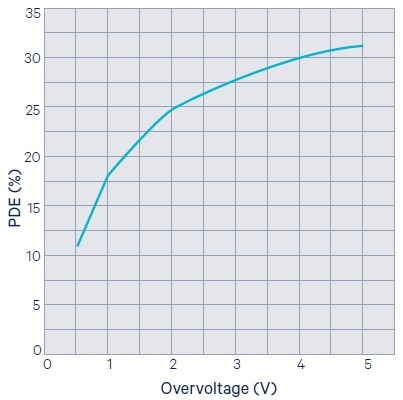Figure 8. Photon detection efficiency (PDE) as a function of the overvoltage. The PDE tends to saturate at high overvoltage because of the saturation of the Pt. 560 nm incident light.Image Credit: First Sensor

Further, the temperature dependence of the breakdown voltage is determined by the structural characteristics of the GM-APDs. An example of the Vbd temperature variation in the SiPMs from First Sensor is shown in Figure 7. For more details, refer to .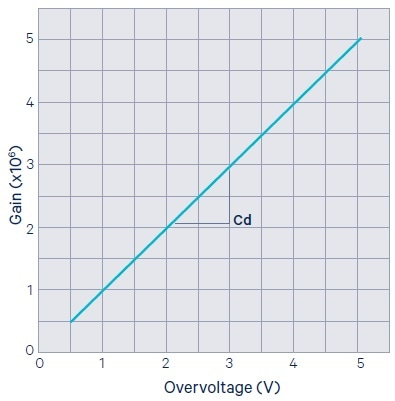Figure 9. SiPM gain as a function of the overvoltage. The slope is the diode capacitance Cd. Image Credit: First Sensor

## Photon Detection Efficiency (PDE)

The photon detection efficiency (PDE) refers to the probability that a photon arriving on the SiPM surface is detected, generating an output pulse. It is defined as the ratio between the number of detected photons over the number of incoming photons.

In terms of device characteristics, the PDE is defined as the product of three factors: quantum efficiency (Qe), triggering probability (Pt) and geometry efficiency (FF):

PDE (Vov) = Qe x Pt (Vov) x FF

Quantum efficiency (Qe) states the probability that a photon impinging on the SiPM is in fact transmitted to the silicon, absorbed in the silicon and finally transformed in an electron/hole pair. Qe is a function of the wavelength and angular incidence of incoming photons. The triggering probability (Pt) is the probability that the generated electron/hole pair successfully starts a self-sustaining avalanche process and thus an output current pulse. Pt is a powerful function of the overvoltage, and it increases with Vov based on the electron and hole ionization rates dependence on the induced electric field . Pt is also wavelength-dependent since the avalanche initiation probability relies on the position inside the GM-APD where the electron/hole pair has been generated and this, in turn, relies on the wavelength of the incoming photon.

The geometry efficiency (or fill-factor, FF) accounts for the fact that each micro-cell in the SiPM has essentially some dead area on its periphery in order to accommodate isolating structures and metal lines for signal routing. Figure 8 depicts a characteristic PDE curve as a function of the overvoltage. The functional dependence of PDE on overvoltage is contained in the Pt term since both Qe and FF are constant factors for a given wavelength. The fill-factor is the key limiting factor in the PDE: for adequately high overvoltage, in fact, both Pt and Qe are closed to 1 and PDE eventually saturates to FF.

By definition, there is 0 < Qe, Pt, FF < 1. Each of its three factors shall be optimized in order to maximize PDE. Qe values as high as 0.98 are attained by means of proper anti-reflecting coating layers deposited over the SiPM active area. Pt is maximized recurring to junction and electric field engineering . Remarkably, the RGB and NUV SiPMs from First Sensor have varied internal junction structure in order to maximize Pt for, respectively, visible light and near ultra violet light ,. To improve cells, fill-factor it is necessary for one to recur to advance lithography techniques with sub-micron feature size and clever device layout .

## Gain

The gain (G) is defined as the number of carriers present in the single-cell current pulse since this refers to the number of carriers produced during the avalanche in response to an absorbed photon. From Figure 3 and relative discussion, the gain is provided by the discharge of Cd from Vbias = (Vbd + Vov ) to Vbd and thus is given by the charge stored on a capacitor of capacitance Cd with a potential difference of Vov, where q is the elementary charge:

G (Vov) = (Cd x Vov) / q

Assuming Cd constant for Vbias > Vbd, gain increases linearly with the overvoltage (Figure 9). Furthermore, G is considered to be practically insensitive to temperature differences since Cd does not vary appreciably with temperature (within a reasonable range of temperatures). At a given bias, any gain shift with temperature has to be qualified to the breakdown voltage shift with temperature. At an overvoltage more than 3 V, the SiPMs from First Sensor show gain differences of less than 1%/°C, thanks to the low temperature coefficient of Vbd (Figure 7).

## Primary Noise (Dark Count Rate)

Noise in SiPMs is represented by spurious output current pulses generated in the absence of light. In silicon, there is a finite probability for carriers (electron and hole) to be produced by thermal agitation. During the quiescent mode, if a hole or an electron originates inside the active region of a GM-APD an avalanche is started (with probability Pt) and an output pulse is observed. This is known as a dark event. The number of dark events per unit time refers to the dark count rate (DCR). In silicon photomultipliers, the thermal generation of carriers doubles almost every 10 °C, and so does the DCR. Furthermore, the DCR scales according to the SiPM area and it is an increasing function of the overvoltage.

A dark event is indistinguishable from a photo-generated one in a single GM-APD. In both cases, the output pulse has 1 p.e. amplitude (Figure 4). In a SiPM, dark events still have 1 p.e. amplitude (neglecting direct optical crosstalk events, disused below), while the useful signal may comprise of more photons impinging on the detector simultaneously producing signals with an amplitude higher than 1 p.e. (see also Figure 5). In this case, it is possible to set a threshold example, 1.5 p.e. to discard the dark events.

The DCR of a SiPM should be specified for a given SiPM size, overvoltage, micro-cell size and temperature. Since the primary noise scales with the silicon area, for a given SiPM technology, the DCR can be provided as Hz (or cps) per unit area, with temperature and overvoltage always specified. In certain cases, it could be useful to have the DCR specified for a particular PDE rather than a specific overvoltage.

## Correlated Noise: Afterpulsing, Optical Crosstalk, ECF

Besides the primary noise, in SiPMs, there are two other sources of noise, that is, afterpulsing (AP) and optical crosstalk (OC). Both OC and AP events originate from an existing current pulse (which can be either a dark-event or a photon-event) and for this reason they are known as correlated noise. The probability of having an OC or AP event intensely depends on the current density in the original avalanche and on the triggering probability Pt. Large micro-cells have large gain, hence an increasing number of carriers flow during the avalanche and thus high OC and AP probability. To contain the correlated noise, it is critical to implement SiPMs with tiny micro-cells .

Afterpulsing is due to the carriers trapped in silicon defects during the avalanche multiplication that are discharged later on during the recharge phase of the GM-APD.

The net effect refers to the observation of a new current pulse on the tail of the original pulse (Figure 10).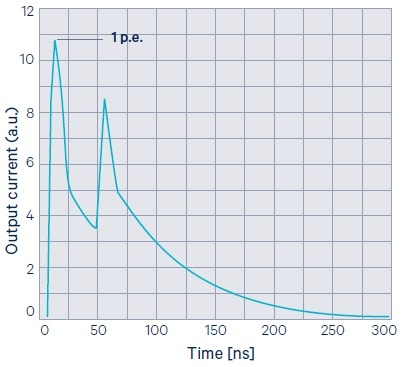Figure 10. Afterpulsing in SiPMs. The same micro-cell fires again during the recharge phase due to a carrier being released from a previously filled silicon trap. Note that the amplitude of the afterpulse is <1 p.e. since Cd has not recharged to Vbias yet when AP occurs. Image Credit: First Sensor

AP probability increases more than linearly with the overvoltage and quadratically with the cell size due to the corresponding increase in the gain. Optical crosstalk includes photons emitted during avalanche multiplication that are re-absorbed in adjacent cells or even in the inactive region of the same cell and triggering additional current pulses. Direct-OC happens when an emitted photon reaches the active region of another cell activating an additional avalanche practically at the same instant of the original avalanche (take into account the speed of light in silicon and the extremely rapid avalanche ignition in GM-APDs).

The result is the double pulse in Figure 11. Delayed-OC instead deals with photons that are re-absorbed in the inactive regions of the SiPM. It is then essential for the generated electron (or hole) to diffuse to the active region of a cell prior to triggering an avalanche. Thus, the correlated pulse has a specific time-delay (in the order of few ns) with respect to the original one (the third signal in Figure 11).

As for AP, in first approximation OP probability increases more than linearly with the overvoltage and quadratically with the cell size. For a more comprehensive treatment of optical crosstalk in SiPMs refer to .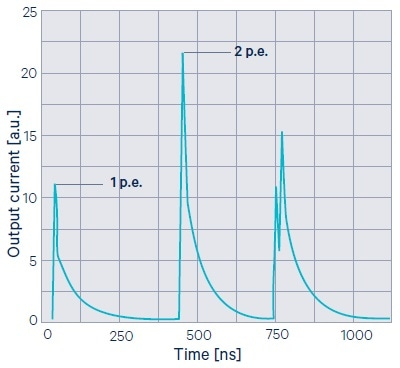Figure 11. Optical crosstalk in SiPMs. From left to right: a single-cell signal (1 p.e.), a direct crosstalk signal (2 p.e.; two cells firing at the same time), and a delayed crosstalk (a second cell fires a few nano seconds after the first one). Image Credit: First Sensor

The effect of both AP and OC is to generate additional charge with respect to the charge related to an original SiPM pulse (photon-event or a dark-event). The SiPM dark current (reverse bias IV characteristics, Vov > 0) is provided by the charge due to primary dark pulses plus the charge due to AP and OC pulses. If no AP and no OC is assumed, then the SiPM dark current at a given Vov comprises of the charge due to primary dark pulses only:

Idark_ideal (Vov) = q x GAIN (Vov) x DCR (Vov)

This is considered to be an “ideal” current since in practice there is continuously a contribution due to AP and OC events. It is possible to model the contribution of AP and OC to the SiPM dark current with an additional term to the ideal SiPM dark current known as excess charge factor (ECF):

Idark_real (Vov) = q x GAIN (Vov) x DCR (Vov) x ECF

Since both Idark_real(Vov) and DCR(Vov) can be precisely measured in laboratory, it is possible to quantify the ECF as:

ECF (Vov) = Idark_real (Vov) / Idark_ideal (Vov)

Figure 12 discusses a typical plot of the ECF as a function of the overvoltage. By definition, in absence of AP and OC, ECF is one. At low overvoltage, AP and OC probabilities are extremely low and ECF is closed to 1.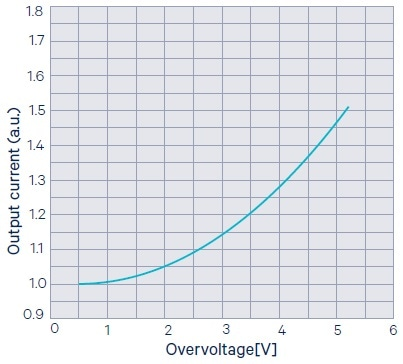Figure 12. The excess charge factor (ECF) in SiMs accounts for the correlated noise (afterpulsing and crosstalk). Its dependence on the overvoltage reflects the compound AP+OC probability dependence on the overvoltage. Image Credit: First Sensor

References

 N. Serra, G. Giacomini, A. Piazza, C. Piemonte, A. Tarolli, N. Zorzi, Experimental and TCAD Study of Breakdown Voltage Temperature Behavior in n+/p SiPMs, in «IEEE TRANSACTIONS ON NUCLEAR SCIENCE», vol. 58, n. 3, 2011, pp. 1233 – 1240

 S. Sze and K. Ng, Physics of Semiconductor Devices, 3rd ed. Hoboken, NJ: Wiley-Interscience, 2006.

 N. Serra, A. Ferri, A. Gola, T. Pro, A. Tarolli, N. Zorzi, C. Piemonte, Characterization of new FBK SiPM technology for visible light detection, in «JOURNAL OF INSTRUMENTATION», vol. 8, n. P03019, 2013

 T. Pro, A. Ferri, A. Gola, N. Serra, A. Tarolli, N. Zorzi, C. Piemonte, New Developments of Near-UV SiPMs at FBK, in «IEEE TRANSACTIONS ON NUCLEAR SCIENCE», vol. 60, n. 3, 2013 , pp. 2247 – 2253

 C. Piemonte, A. Ferri, A. Gola, T. Pro, N. Serra, A. Tarolli, N. Zorzi, Characterization of the First FBK High- Density Cell Silicon Photomultiplier Technology, in «IEEE TRANSACTIONS ON ELECTRON DEVICES», vol. 60, n. 8, 2013, pp. 2567 – 2573

 C. Piemonte, A. Ferri, A. Gola, A. Picciotto, T. Pro, N. Serra, A. Tarolli, N. Zorzi, Development of an automatic procedure for the characterization of silicon photomultipliers, Conference Record of NSS/MIC 2012, IEEE, 2012, pp. 428-432

 A. Gola, A. Tarolli, C. Piemonte, SiPM Cross-talk Amplification due to Scintillator Crystal: Effects on Timing Performance, Conference Record of NSS/MIC 2012, IEEE, 2012 , pp. 421-423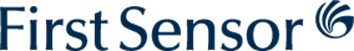This information has been sourced, reviewed and adapted from materials provided by First Sensor AG.

## Citations

• APA

First Sensor AG. (2020, May 18). What are Silicon Photomultipliers (SiPMs)?. AZoSensors. Retrieved on June 02, 2020 from https://www.azosensors.com/article.aspx?ArticleID=865.

• MLA

First Sensor AG. "What are Silicon Photomultipliers (SiPMs)?". AZoSensors. 02 June 2020. <https://www.azosensors.com/article.aspx?ArticleID=865>.

• Chicago

First Sensor AG. "What are Silicon Photomultipliers (SiPMs)?". AZoSensors. https://www.azosensors.com/article.aspx?ArticleID=865. (accessed June 02, 2020).

• Harvard

First Sensor AG. 2020. What are Silicon Photomultipliers (SiPMs)?. AZoSensors, viewed 02 June 2020, https://www.azosensors.com/article.aspx?ArticleID=865.

## Tell Us What You Think